# 3,82 M 4el OWA 8,42 dBIFor:             DX portion 11 meter band
Antenna Type:   4el OWA-YAGI
optimum wide band array
Designed by:    HPSD version 1.01 Nov 2005
Boom length:     3,82 Meter
Gain:         8,42 dBI (@27,555 Mhz)
FB            <22 dB
FR            <22 dB
Impedance:         50 ohms, direct fed
SWR below 1:1.1     > 350 Khz.
SWR below 2:1    > 1700 Khz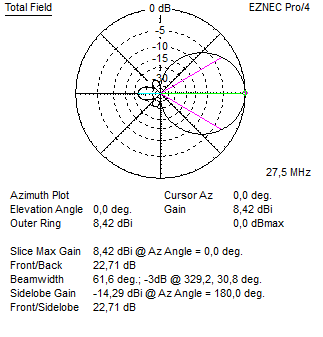Above the azimuth plot. 8,42 dBI gain.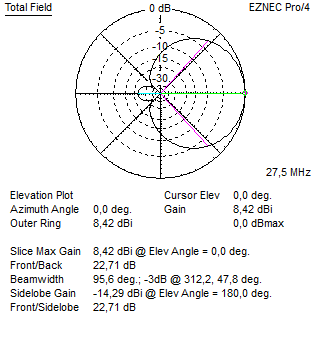Above the freespace elevation plot.

Below the predicted SWR plot: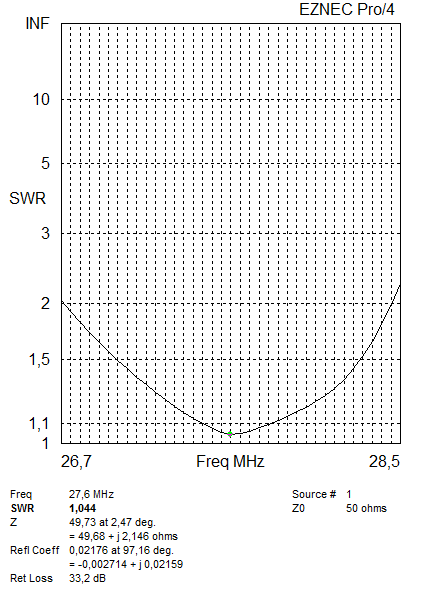3 meter long 22 mm tubes (center piece) inserted with 18mm (end tips).

Distances:

Reflector: Director 1 = 1,834 meter

Reflector: Director 2 = 3,82 Meter

Length:

Reflector = 2x 2,736 Meter (5,472 Meter)

Radiator = 2x 2,657 Meter  (5,314 Meter)

Director 1 = 2x 2,523 Meter  (5,046 Meter)

Director 2 = 2×2,4062 Meter  (4,813 Meter)

4 elements OWA using INCH ELEMENT DIAMETER: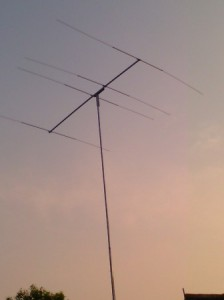A home made 4el OWA using inches at : 91SD/OC075    (91SD750).

Thank you for sending the picture Itji !

For:             DX portion 11 meter band
Antenna Type:   4el OWA-YAGI
optimum wide band array
Designed by:    HPSD version 1.01 Nov 2005
Boom length:     3,82 Meter
Gain:         8,32 dBI (@27,555 Mhz)
FB            <22 dB
FR            <22 dB
Impedance:         50 ohms, direct fed
SWR 1:1.1     < 350 Khz.
SWR  2:1    < 1700 Khz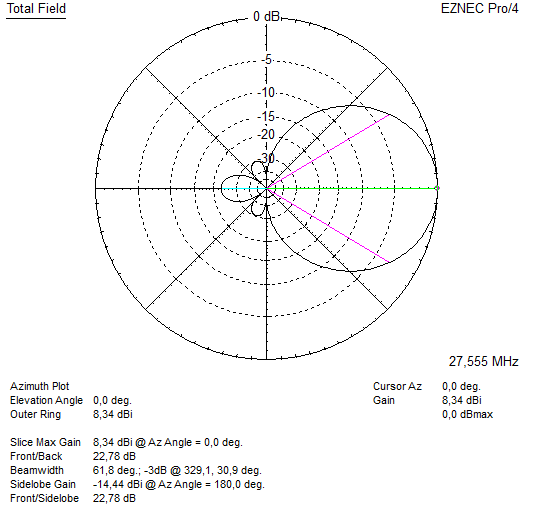Above the azimuth plot. 8,34 dBI gain.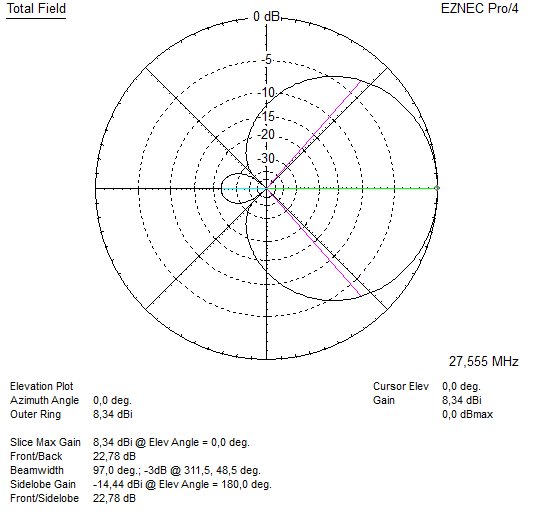Above the freespace elevation plot.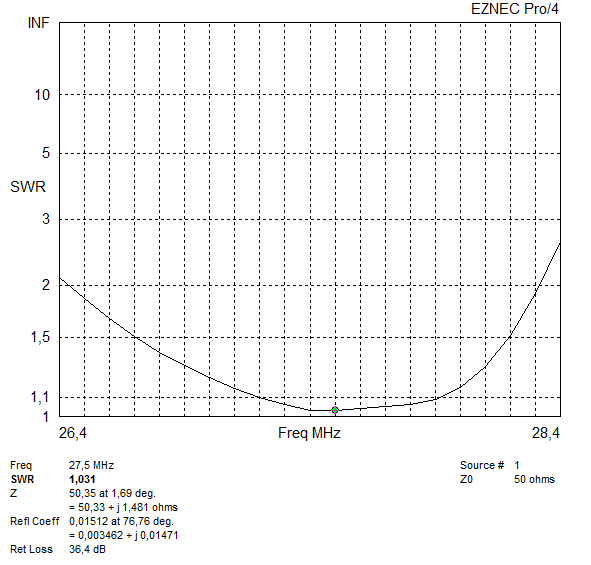Below the predicted SWR plot:

5/8 inch and 1/2 inch tubing.

The 5/8 inch tubing is 3 meters long the 1/2 inch tubing are inserted on both ends to complete the element length.

Distances:

Reflector: Director 1 = 1,920meter

Reflector: Director 2 = 3,82 Meter

Length:

Reflector = 2x 2,750 Meter (5,50 Meter)

Radiator = 2x 2,700Meter  (5,40 Meter)

Director 1 = 2x 2,560 Meter  (5,12 Meter)

Director 2 = 2×2,435 Meter  (4,87 Meter)